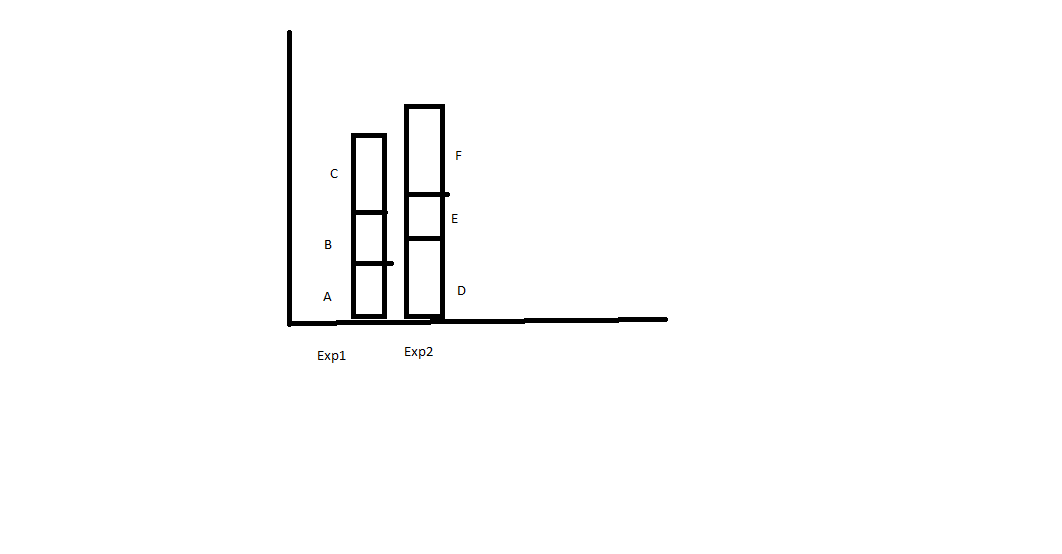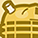# New to Qlik Sense

If you’re new to Qlik Sense, start with this Discussion Board and get up-to-speed quickly.

Announcements
Our May 2021 end-to-end product release from Data Integration to Data Analytics is out! READ DETAILS
cancel
Showing results for
Did you mean:Contributor III

## Qlik Sense Stacked Bar chart without Dimensions

Hi Team,

I have a scenario like given below

Dimension - No Dimension

Expression1 - Sum(A)+Sum(B+sum(C)

Expression 2 - sum(D) +sum(E)+sum(F)

I have to show the value in Stacked Bar chart as Attached1 Solution

Accepted SolutionsPartner

Dimension:

ValueList('exp1','exp2')

Measure 1:

if(ValueList('exp1','exp2')='exp1',sum(A),sum(D))

Measure 2:

if(ValueList('exp1','exp2')='exp1',sum(B),sum(E))

Measure 3:

if(ValueList('exp1','exp2')='exp1',sum(C),sum(F))

Result:3 RepliesPartner

Dimension:

ValueList('exp1','exp2')

Measure 1:

if(ValueList('exp1','exp2')='exp1',sum(A),sum(D))

Measure 2:

if(ValueList('exp1','exp2')='exp1',sum(B),sum(E))

Measure 3:

if(ValueList('exp1','exp2')='exp1',sum(C),sum(F))

Result:Contributor III
Author

Hi Omar,

Thanks.Contributor II

Hi Omar,

I have the same question but with 3 different dimension.

Can you give example using the same example here but add 'exp3'?

Really appreciate if you can help.

Thanks.